### Home > A2C > Chapter Ch4 > Lesson 4.1.2 > Problem4-31

4-31.
1. Simplify each expression without using a calculator. Remember that to simplify expressions with radicals, you can remove perfect square factors. Part (a) below is provided as an example. Homework Help ✎

1.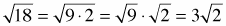2.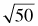3.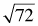4.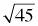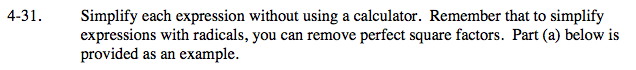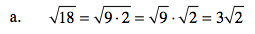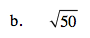What square number is a factor of 50?

$5\sqrt{2}$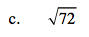Use the same method as in parts (a) and (b).

To simplify completely you need to find the largest square number that is a factor of 72.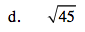Use the same method as in parts (a) and (b).